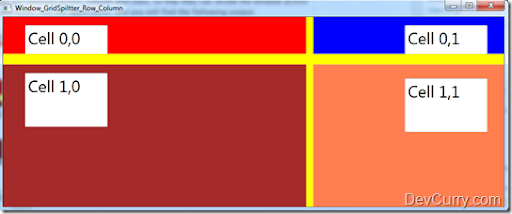# Using GridSplitter in Windows Presentation Foundation (WPF)

 Tweet

WPF provides various control for enhancing User experience (UX). One of the very useful category of controls is Panel controls viz Grid, WrapPanel, DockPanel, StackPanel etc. All these controls are used for effective layout. Along with these controls, to resize UI elements at runtime, the ‘GridSplitter’ is provided which splits the UI in horizontal and vertical direction i.e. across rows and columns. The XAML code snippet shown below provides an example of using the GridSplitter.

`<Window x:Class="WPF40_GridSplitter.Window_GridSpiltter_Row_Column"        xmlns="http://schemas.microsoft.com/winfx/2006/xaml/presentation"        xmlns:x="http://schemas.microsoft.com/winfx/2006/xaml"        Title="Window_GridSpiltter_Row_Column" Height="363" Width="875">    <Grid x:Name="grdMain">        <Grid.RowDefinitions>            <RowDefinition Height="147*" />            <RowDefinition Height="18*" />            <RowDefinition Height="159*" />        </Grid.RowDefinitions>        <Grid.ColumnDefinitions>            <ColumnDefinition Width="246*" />            <ColumnDefinition Width="12*" />            <ColumnDefinition Width="595*" />        </Grid.ColumnDefinitions>        <Grid Background="Red" Grid.Column="0">            <TextBox Height="92" HorizontalAlignment="Left"                     Margin="38,14,0,0" Name="textBox1"                     VerticalAlignment="Top" Width="141"                     Text="Cell 0,0" FontSize="28" />        </Grid>        <GridSplitter Grid.Row="0" Grid.Column="1" Background="Yellow"                       HorizontalAlignment="Stretch"                    VerticalAlignment="Stretch" Width="Auto" Height="Auto"                      Grid.RowSpan="3" ResizeDirection="Columns">               </GridSplitter>        <Grid Grid.Row="0" Grid.Column="2" Background="Blue">            <TextBox FontSize="28" Height="92" HorizontalAlignment="Left"                     Margin="157,14,0,0" Name="textBox2" Text="Cell 0,1"                     VerticalAlignment="Top" Width="141" />        </Grid>        <GridSplitter Grid.Row="1" Grid.Column="0" Background="Yellow"                HorizontalAlignment="Stretch"  VerticalAlignment="Stretch"                 Width="Auto" Height="Auto" Grid.ColumnSpan="3"               ResizeDirection="Rows"></GridSplitter>        <Grid Grid.Row="2" Grid.Column="0" Background="Brown">            <TextBox FontSize="28" Height="92" HorizontalAlignment="Left"                   Margin="38,14,0,0" Name="textBox3"                   Text="Cell 1,0" VerticalAlignment="Top" Width="141" />        </Grid>        <Grid Grid.Row="2" Grid.Column="2" Background="Coral">            <TextBox FontSize="28" Height="92" HorizontalAlignment="Left"                   Margin="157,23,0,0" Name="textBox4"                   Text="Cell 1,1" VerticalAlignment="Top" Width="141" />        </Grid>    </Grid></Window>`

If you observe the XAML code, the main grid ‘grdMain’ is divided into 3 rows and 3 columns. There are four Grid elements in various cells of grdMain. There are two GridSplitters used for resizing across columns and rows respectively. These are with ‘Yellow’ background and span across Column no. 2, for column resize and Row no. 2, for row resize. Both these GridSplitters are spanned across column and rows, so that they can divide the window across Columns and Rows. Run the application, and you will find the following output.

After resizing rows and columns at runtime